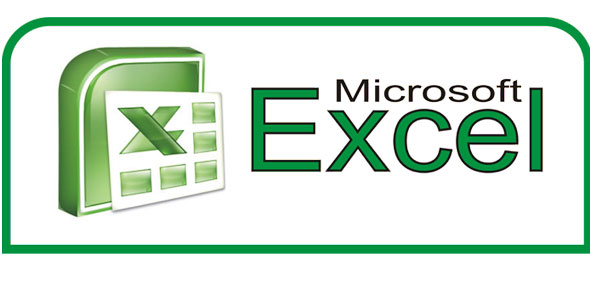# Excel Vocabulary

5 Questions | Total Attempts: 547SettingsVocabulary test for Microsoft Excel

Related Topics
• 1.
What is Microsoft Excel?
• A.

A spreadsheet application tool that analyses data in a table format using formulas

• B.

A multimedia presentation in which slides are used to create that presentation

• C.

A word processing program

• D.

A relational database management system designed for use on personal computers.

• 2.
• A.

Vertical spaces and headed with letters

• B.

A mathematical equation typed into a cell

• C.

The exact location of a specific cell

• D.

Function allowing the user to select a number of cells to create one large cell

• 3.
What is an active cell?
• A.

The toolbar on the Microsoft Excel window that shows the text or equations assigned to cells

• B.

The cell in a spreadsheet that is slected. It has a dark border around the information.

• C.

A spreadsheet application tool that analyzes data in a table format using formulas.

• D.

Labels located at the bottom of the workbook window indicating the worksheets.

• 4.
What is a row?
• A.

Is the horizontal group of cells. They are named by numbers.

• B.

Is the vertical group of cells. They are named by letters.

• 5.
What is the name box?
• A.

Function that allows the user to change the value of a cell to a percent.

• B.

A single sheet contained in an Excel workbook.

• C.

Function that changed the contents of a cell to currency. Adds a dollar sign and decimal places.

• D.

Displays the cell reference, which is the location of the active cell in the worksheet. it is located ont he left side of the formula bar.